#### Probability space

A probability space is a three-tuple,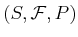, in which the three components are

1. Sample space: A nonempty set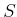called the sample space, which represents all possible outcomes.
2. Event space: A collection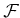of subsets of, called the event space. Ifis discrete, then usually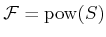. Ifis continuous, thenis usually a sigma-algebra on, as defined in Section 5.1.3.
3. Probability function: A function,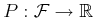, that assigns probabilities to the events in. This will sometimes be referred to as a probability distribution over.
The probability function,, must satisfy several basic axioms:
1.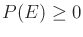for all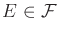.
2.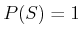.
3.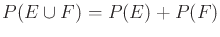if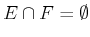, for all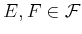.
Ifis discrete, then the definition ofover all ofcan be inferred from its definition on single elements ofby using the axioms. It is common in this case to write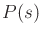for some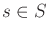, which is slightly abusive because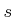is not an event. It technically should be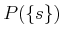for some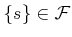.

Example 9..4 (Tossing a Die)   Consider tossing a six-sided cube or die that has numbersto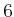painted on its sides. When the die comes to rest, it will always show one number. In this case,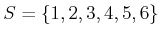is the sample space. The event space is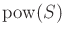, which is all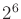subsets of. Suppose that the probability function is assigned to indicate that all numbers are equally likely. For any individual,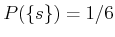. The events include all subsets so that any probability statement can be formulated. For example, what is the probability that an even number is obtained? The event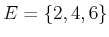has probability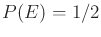of occurring.The third probability axiom looks similar to the last axiom in the definition of a measure space in Section 5.1.3. In fact,is technically a special kind of measure space as mentioned in Example 5.12. Ifis continuous, however, this measure cannot be captured by defining probabilities over the singleton sets. The probabilities of singleton sets are usually zero. Instead, a probability density function,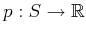, is used to define the probability measure. The probability function,, for any eventcan then be determined via integration: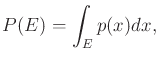(9.3)

in which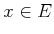is the variable of integration. Intuitively,indicates the total probability mass that accumulates over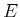.

Steven M LaValle 2020-08-14